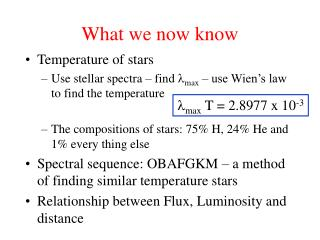Download PresentationWhat we now know

What we now know - PowerPoint PPT Presentation

What we now know. l max T = 2.8977 x 10 -3. Temperature of stars Use stellar spectra – find l max – use Wien’s law to find the temperature The compositions of stars: 75% H, 24% He and 1% every thing else Spectral sequence: OBAFGKM – a method of finding similar temperature starsI am the owner, or an agent authorized to act on behalf of the owner, of the copyrighted work described.
Download PresentationWhat we now know

Download Policy: Content on the Website is provided to you AS IS for your information and personal use and may not be sold / licensed / shared on other websites without getting consent from its author.While downloading, if for some reason you are not able to download a presentation, the publisher may have deleted the file from their server.

- - - - - - - - - - - - - - - - - - - - - - - - - - E N D - - - - - - - - - - - - - - - - - - - - - - - - - -
Presentation Transcript
1. What we now know lmax T = 2.8977 x 10-3 • Temperature of stars • Use stellar spectra – find lmax – use Wien’s law to find the temperature • The compositions of stars: 75% H, 24% He and 1% every thing else • Spectral sequence: OBAFGKM – a method of finding similar temperature stars • Relationship between Flux, Luminosity and distance

2. Stellar luminosity If we combine the flux measurement F and the distance measurement d for a star, then we can find its luminosity Why! You measure its parallax me old befuddled chum So, how do we find the distance to a star? A fundamental star property or,

3. Distances from Angles The key to finding distance is to measure position angles from two separate locations 2 a X D 1 With X and a being measured river Elementary my dear Watson

4. Distances to the stars Direct method: stellar parallax no assumptions need be made about the star Observations: measure the apparent shift in the position of a near-by star over a 6 month time interval The point! The Earth moves half way around its orbit in this time interval

5. Stellar Parallax – an apparent shift in the position of a star due to Earth’s motion about the Sun During a 6 month time interval the nearby star moves through a measurable angle WRT the much more distant background stars

6. And the geometry says: Distant stars Obs. 2 Earth’s orbit Distance, d 1 AU P Sun Near-by star Obs. 1 Idea is that observations give P the angle of parallax

7. Observations reveal the 6-month motion of the near-by star Time from Obs. 1 to Obs. 2 = 6 months View from Obs. 1 View from Obs. 2 Measure: Angular shift = 2 P Note: the further a star is away, so the smaller is the angle of parallax

8. Parallax motion in the direction of Orion

9. Trigonometry: Tan(P) = 1 AU / distance (d) The clever bit: for small angles Tan(P)  P(radians) P(radians) = (2 p / 360) P(degrees) P(arcsec.) = P(degrees) x 3600 Result: d = 206,265 AU / P(arcsec.) WTF See triangle handout purely from numerical conversion terms

10. Notes & definition: if P = 1 second of arc, then d = 206,265 AU Hence: if the observed angle of parallax is 1 arc second the distance is defined to be 1 parsec so the conversion factor is 1 parsec = 206,265 AU Final result: d(parsec) = 1 / P(arcsec.)  OOTETK

11. Word origins Parsec The concatenation of parallax and arc second 1 parsec is the distance to an object with a parallax of 1 arc second And doing the Kessel Run in under 12 parsecs just doesn’t make sense – boast you too much me think Han Solo – and fail you Astronomy 101

12. Proxima Centauri Robert Innis – discovered Proxima in 1915 Nearest star to the Solar System and Sun Observed parallax shift on the sky over a six month time interval is Angle = 1.5377 arc seconds = 0.00042714 degrees = 2 x P Proper motion over 25 years Angle of parallax = P = 1.5377 / 2 = 0.76885 arc seconds Distance to Proxima = 1 / P = 1/0.76885 = 1.301 parsecs

13. Let’s get Sirius Distance to Sirius = 8.6 light years What is its angular shift on the sky over a six month interval ?

14. Given: d(Sirius) = 8.6 light years = 2.64 pc So, from Parallax formula (after algebra switch) And hence 6 month angular shift = 2 x P = 0.758 arcsec (equivalent to 2 x 10-4 degrees = human hair at 28-m) Unit conversion Oh! To be far from Azkaban and doing astronomy 101

15. Parallax ain’t easy Parallax shifts are fractions of an arc second ground based limits: P > 0.05 arcsec. (d < 20 pc) space based limits: P > 0.002 arcsec. (d < 500 pc) Beyond about 500 pc must use indirect methods Find and calibrate “standard candles” Definition:Objects of known luminosity (e.g. sun-like stars)

16. First parallax numbers (1837) No need to copy table (Sun-like star)

17. All systems are go…. Calibration step: Stars with measured flux and distance d and spectral type Calculate temperature and luminosity Calibrate spectral type with luminosity = standard candle Once parallax too small to measure Find distance from spectral type - luminosity calibration We can now find the distance to any star in the observable universe – cool  from spectral type calibration measured by parallax